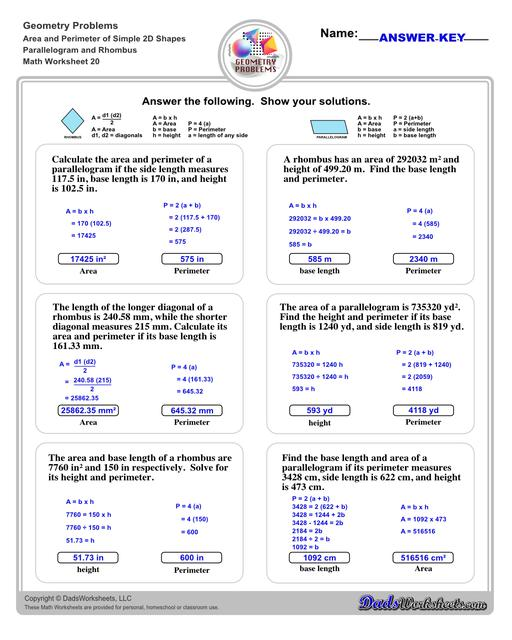Math Worksheets

### Worksheet News

PLEASE GO BACK AND USE THE BIG BLUE 'PRINT' BUTTON ON THE PAGE TO PRINT THE WORKSHEET CORRECTLY!Sorry for the trouble! The browser won't print the embedded worksheet PDF directly using the normal 'Print' command in the file menu, so you need to click the big 'Print' button to send just the worksheet and not the surrounding page to the printer.

# Math Worksheets: Area and Perimeter : Area And Perimeter Of Simple 2d Shapes Parallelogram And Rhombus V4## Math Worksheets: Area and Perimeter: Area And Perimeter Of Simple 2d Shapes Parallelogram And Rhombus V4

Property Value
DescriptionThe area and perimeter worksheets on this page start with requiring students to calculate area and perimeter of basic shapes such as triangles, squares, circles and ellipses. Additional worksheets with compound shapes require students to calculate missing dimensions and use problem solving skills and strategies to calculate area and perimeter. Area And Perimeter Of Simple 2d Shapes Parallelogram And Rhombus V4
Resource TypeWorksheet